## MATHEMATICS NOTES FORM 2 TOPIC 9:TRIGONOMETRY

### MATHEMATICS NOTES FORM 2 TOPIC 9:TRIGONOMETRYThursday, June 18, 2020

MATHEMATICS NOTES FORM 2 TOPIC 9: TRIGONOMETRY

UNAWEZA JIPATIA NOTES ZETU KWA KUCHANGIA KIASI KIDOGO KABISA:PIGA SIMU:0787237719

## MATHEMATICS NOTES FORM 2 TOPIC 9:TRIGONOMETRY

TRIGONOMETRY

Do you want to learn the relationships involving lengths and Angles of right-angled triangle? Here, is where you can learn.

Trigonometry is all about Triangles. In this chapter we are going to deal with Right Angled Triangle. Consider the Right Angled triangle below: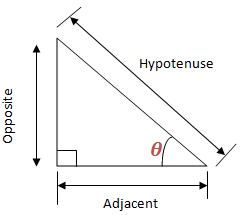The sides are given names according to their properties relating to the Angle .
Opposite side is opposite the Angle
Hypotenuse side is the longest side

Sine, Cosine and Tangent of an Angle using a Right Angled Triangle
Define sine, cosine and tangent of an angle using a right angled triangle
Trigonometry is good at finding the missing side or Angle of a right angled triangle. The special functions, sine, cosine and tangent help us. They are simply one of a triangle divide by another. See similar triangles below: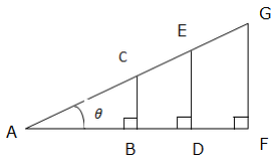The ratios of the corresponding sides are: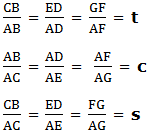Where by t, c and s are constant ratios called tangent (t), cosine (c) and sine (s) of Angle respectively.
The right-angled triangle can be used to define trigonometrical ratios as follows:The short form of Tangent is tan, that of sine is sin and that of Cosine is cos.
The simple way to remember the definition of sine, cosine and tangent is the word SOHCAHTOA. This means sine is Opposite (O) over Hypotenuse (H); cosine is Adjacent (A) over Hypotenuse (H); and tangent is Opposite (O) over Adjacent (A). Or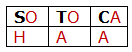Example 1
Given a triangle below, find sine, cosine and Tangent of an angle indicated.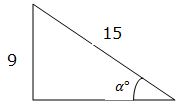Solution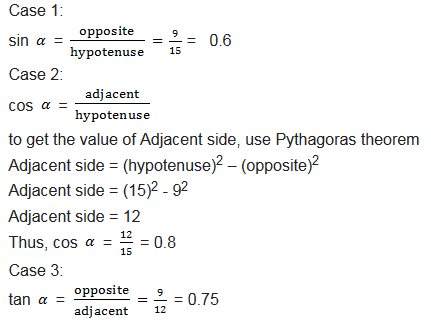Example 2
Given that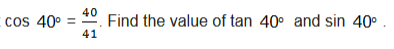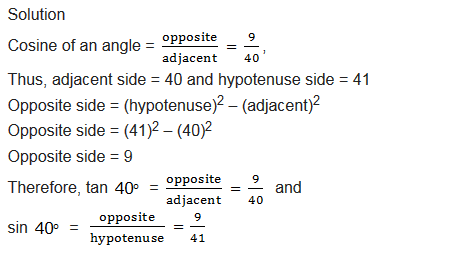Angles of Elevation and Angles of Depression
Demonstrate angles of elevation and angles of depression
Angle of Elevation of an Object as seen by an Observer is the angle between the horizontal and the line from the Object to the Observer’s aye (the line of sight). See the figure below for better understanding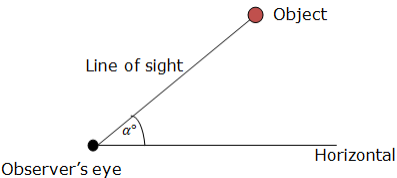The angle of Elevation of the Object from the Observer is α0.
Angle of depression of an Object which is below the level of Observer is the angle between the horizontal and the Observer’s line of sight. To have the angle of depression, an Object must be below the Observer’s level. Consider an illustration below: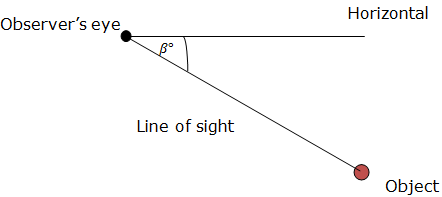The angle of depression of the Object from the Object is β0
Problems involving Angles of Elevation and Angles of Depression
Solve Problems involving angles of elevation and angles of depression
Example 5
From the top of a vertical cliff 40 m high, the angle of a depression of an object that is level with the base of the cliff is350. How far is the Object from the base of the cliff?
Solution
We can represent the given information in diagram as here below: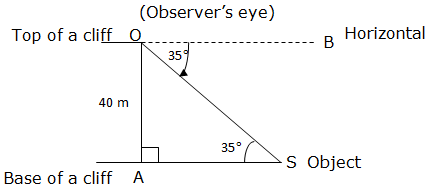Angle of depression = 350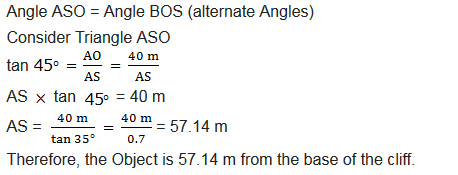Exercise 1
1. Use trigonometric tables to find the following:
1. cos 38.250
2. sin 56.50
3. tan 750
2. Use trigonometrical tables to find the value of x in the following problems.
1. sin x0 = 0.9107
2. tan x0 = 0.4621
3. Find the height of the tower if it casts a shadow of 30 m long when the angle of elevation of the sun is380.
4. The Angle of elevation of the top of a tree of one point from east of it and 56 m away from its base is250. From another point on west of the tree the Angle of elevation of the top is500. Find the distance of the latter point from the base of the tree.
5. A ladder of a length 15m leans against a wall and make an angle of300with a wall. How far up the wall does it reach?

MATHEMATICS NOTES FORM 2 TOPIC 9:TRIGONOMETRY
4/ 5
Oleh